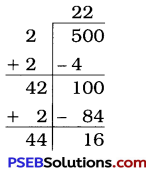# PSEB 8th Class Maths Solutions Chapter 6 Squares and Square Roots Ex 6.4

Punjab State Board PSEB 8th Class Maths Book Solutions Chapter 6 Squares and Square Roots Ex 6.4 Textbook Exercise Questions and Answers.

## PSEB Solutions for Class 8 Maths Chapter 6 Squares and Square Roots Ex 6.4

1. Find the square root of each of the following numbers by Division method.

Question (i).
2304
Solution: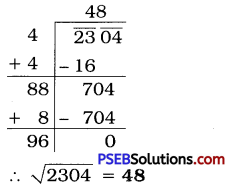Question (ii).
4489
Solution: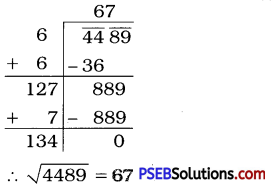Question (iii).
3481
Solution: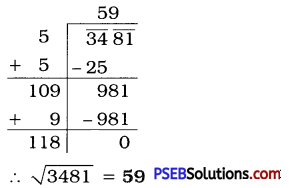Question (iv).
529
Solution: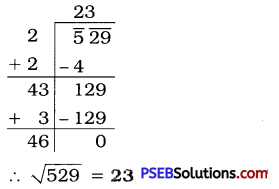Question (v).
3249
Solution: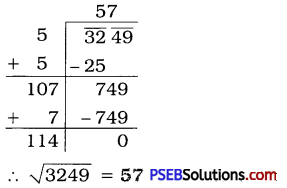Question (vi).
1369
Solution: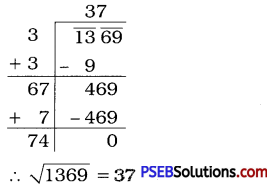Question (vii).
5776
Solution: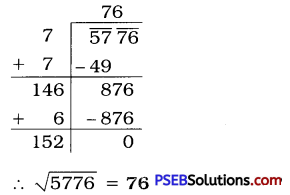Question (viii).
7921
Solution: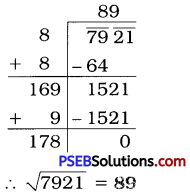Question (ix).
576
Solution: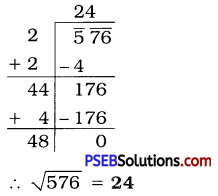Question (x).
1024
Solution: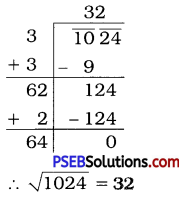Question (xi).
3136
Solution: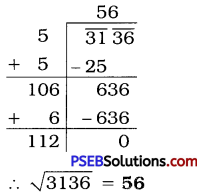Question (xii).
900
Solution: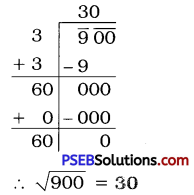2. Find the number of digits in the square root of each of the following numbers (without any calculation).

Question (i).
64
Solution:
Here, number of digits, n = 2
(Which is an even number.)
∴ Number of digits in the square root of 64 = $$\frac{n}{2}=\frac{2}{2}$$ = 1

Question (ii).
144
Solution:
Here, number of digits, n = 3
(Which is an odd number.)
∴ Number of digits in the square root of 144 = $$\frac{n+1}{2}=\frac{3+1}{2}$$
= $$\frac {4}{2}$$
= 2Question (iii).
4489
Solution:
Here, number of digits, n = 4
(Which is an even number.)
∴ Number of digits in the square root of 4489 = $$\frac{n}{2}=\frac{4}{2}$$
= 2

Question (iv).
27225
Solution:
Here, number of digits, n = 5
(Which is an odd number.)
∴ Number of digits in the square root of 27225 = $$\frac{n+1}{2}=\frac{5+1}{2}$$
= $$\frac {6}{2}$$
= 3

Question (v).
390625
Solution:
Here, number of digits, n = 6
(Which is an even number.)
∴ Number of digits in the square root of 390625 = $$\frac{n}{2}=\frac{6}{2}$$
= 3

3. Find the square root of the following decimal numbers.

Question (i).
2.56
Solution: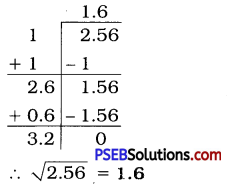Here, number of decimal places are two.
∴ The number of decimal places in square root should be one.

Question (ii).
7.29
Solution: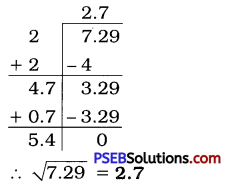Here, number of decimal places are two.
∴ The number of decimal places in square root should be one.Question (iii).
51.84
Solution: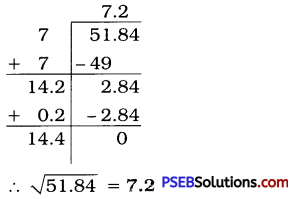Here, number of 51.84 decimal places are two.
∴ The number of decimal places in square root should be one.

Question (iv).
42.25
Solution: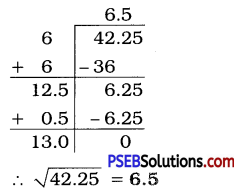Question (v).
31.36
Solution: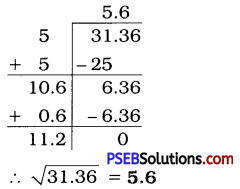4. Find the least number which must be subtracted from each of the following numbers so as to get a perfect square. Also find the square root of the perfect square so obtained.

Question (i).
402
Solution: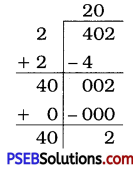Here, the remainder is 2. It shows that 202 is less than 402 by 2.
So, to get a perfect square, 2 must be subtracted from given number.
∴ Required perfect square number = 402 – 2 = 400
$$\sqrt{400}$$ = 20Question (ii).
1989
Solution: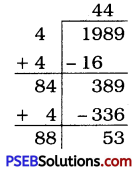Here, the remainder is 53. It shows that 442 is less than 1989 by 53.
So, to get a perfect square, 53 must be subtracted from the given number.
∴ Required perfect square number = 1989 – 53 = 1936
$$\sqrt{1936}$$ = 44

Question (iii).
3250
Solution: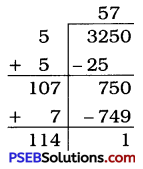Here, the remainder is 1. It shows that 572 is less them 3250 by 1.
So, to get a perfect square, 1 must be subtracted from the given number.
∴ Required perfect square number = 3250 – 1 = 3249
$$\sqrt{3249}$$ = 57

Question (iv).
825
Solution: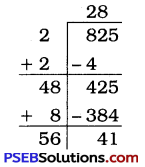Here, the remainder is 41. It shows that 282 is less than 825 by 41.
So, to get a perfect square, 41 must be subtracted from the given number.
∴ Required perfect square number = 825 – 41 = 784
$$\sqrt{784}$$ = 28

Question (v).
4000
Solution: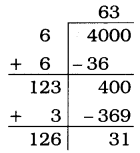Here, the remainder is 31. It shows that 632 is less than 4000 by 31.
So, to get a perfect square, 31 must be subtracted from the given number.
∴ Required perfect square number = 4000 – 31 = 3969
$$\sqrt{3969}$$ = 635. Find the least number which must be added to each of the following numbers so as to get a perfect square. Also find the square root of the perfect square so obtained.

Question (i).
525
Solution: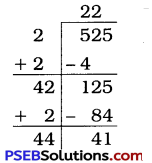Here, the remainder is 41.
525 > 222,
and the number next to 22 is 23.
232 = 529.
∴ The required number to be added
= 232 – 525
= 529 – 525 = 4
Now, 525 + 4 = 529
$$\sqrt{529}$$ = 23
Thus, 4 is the least number which must be added to 525 to get a perfect square.

Question (ii).
1750
Solution: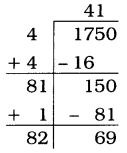Here, the remainder is 69.
1750 > 412,
and the number next to 41 is 42.
422 = 1764.
∴ The required number to be added = 422 – 1750
= 1764 – 1750
= 14
Now, 1750 + 14 = 1764
$$\sqrt{1764}$$ = 42
Thus, 14 is the least number which must be added to 1750 to get a perfect square.

Question (iii).
252
Solution: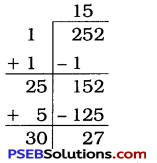Here, the remainder is 27.
252 > 152,
and the number next to 15 is 16.
162 = 256.
∴ The required number to be added = 162 – 252
= 256 – 252
= 4
Now, 252 + 4 = 256
$$\sqrt{256}$$ = 16
Thus, 4 is the least number which must be added to 252 to get a perfect square.

Question (iv).
1825
Solution: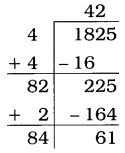Here, the remainder is 61.
1825 > 422,
and the number next to 42 is 43.
432 = 1849.
∴ The required number to be added 432 – 1825
= 1849 – 1825
= 24
Now, 1825 + 24 = 1849
$$\sqrt{1849}$$ = 43
Thus, 24 is the least number which must be added to 1825 to get a perfect square.Question (v).
6412
Solution: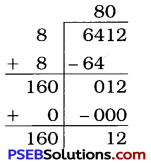Here, the remainder is 12.
6412 > 802,
and the number next to 80 is 81.
812 = 6561.
∴ The required number to be added = 812 – 6412
= 6561 – 6412
= 149
Now, 6412 + 149 = 6561
$$\sqrt{6561}$$ = 81
Thus, 149 is the least number which must be added to 6412 to get a perfect square.

6. Find the length of the side of a square whose area is 441 m2.
Solution:
Let the side of a square be x m.
Area of a square = x × x = x2
Area of a square = 441 (given)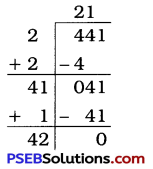∴x2 = 441
∴ x = $$\sqrt{441}$$
∴ x = 21
Thus, the length of the side of the square is 21 m.

7. In a right triangle ABC, ∠B = 90° :
(a) If AB = 6 cm, BC = 8 cm, find AC.
(b) If AC = 13 cm, BC = 5 cm, find AB.
Solution:
[Note: In a right triangle, the longest side is called the hypotenuse. The square of the hypotenuse is equal to the sum of the squares of the remaining two sides.]
Let us use this theorem here.
(a) Here, ∠B = 90°, AB = 6 cm, BC = 8 cm
In ΔABC, AC is hypotenuse.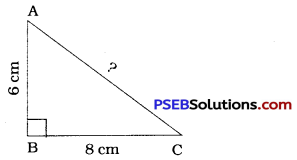AC2 = AB2 + BC2
= (6)2 + (8)2
= 36 + 64
=100
∴ AC = $$\sqrt{100}$$
= 10cm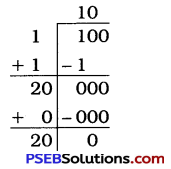(b) Here, ∠B= 90°, AC = 13cm, BC = 5cm
In ΔABC, AC Is hypotenuse.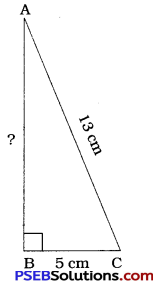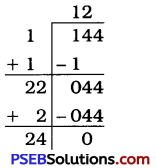AC2 = AB2 + BC2
∴ (13)2 = AB2 + (5)2
∴ 169 = AB2 + 25
∴ AB2 = 169 – 25
= 144
∴ AB = $$\sqrt{144}$$
= 12 cm8. A gardener has 1000 plants. He wants to plant these in such a way that the number of rows and the number of columns remain same. Find the minimum number of plants he needs more for this.
Solution:
Total number of plants = 1000
The number of plants in a row = The number of plants in a column
Let the number of plants planted in a row be x.
So, the number of plants planted in a column is x.
∴ Total plants = x × x = x2
∴ x2 > 1000
∴ x > $$\sqrt{1000}$$
Here, the remainder is 39.
(31)2 < 1000
The next square number would be 32.
322 = 1024
∴ The number of plants required to be added = 1024 – 1000
= 24
Thus, 24 more plants needed.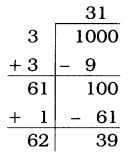9. There are 500 children in a school. For a P.T. drill they have to stand in such a manner that the number of rows is equal to number of columns. How many children would be left out in this arrangement?
Solution:
Total number of children in a school = 500
The number of rows = The number of columns
Let the number of children in a row be x.
So, the number of children in a column is x.
Total number of children = x × x = x2.
∴ x2 < 500
∴ x < $$\sqrt{500}$$
Here, the remainder is 16.
500 > 222
∴ 500 > 484
500 – 484 = 16
Thus, 16 children would be left out in this arrangement.